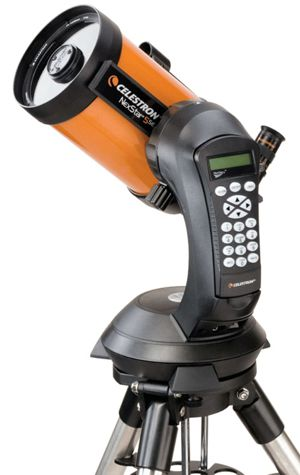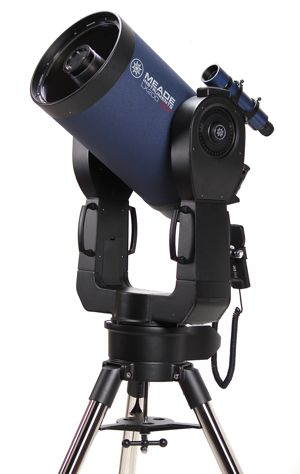# Theory## Introduction

The magnitude limit formula, Lmag = 2 + 5 log(DO) appears to be something that you can't simply calculate in your head. But... if you can find log(DO) in your head to within a tenth (i.e. within 0.1) you can then estimate the magnitude limit within a half-magnitude.

Turns out this is something you can do, usually in less than 15 seconds. Amuse your friends! Amaze your pets! Baffle your colleagues! Best of all... know what to expect from your telescope...

## What to Know

1. Fundamentals of Logarithms
1. What's a Log?
• The logarithm of a number is the exponent to which 10 is raised to produce that number.
• The logarithm of a number is written log(number)
2. Integer Examples
• log(10) = 1, since 101 = 10
• log(100) = 2, since 102 = 100
• log(1000) = 3, since 103 = 1000
• log(1) = 0, since 100 = 1
3. Non-integer Examples
Non-integer logarithms result when the number is not an even power of 10
• log(2) = 0.3
• log(3) = 0.477
4. Operations with Logarithms
• When you multiply two numbers to get a result, you add their logarithms to get the logarithm of the result.
• Obvious example: 10*10 = 100; log(10) = 1, and 1+1 = 2, which is log(100)
• Less obvious example: 2*3 = 6; so log(2)+log(3) = 0.3+0.477 = 0.777 = log(6)
• When you divide two numbers to get a result, you subtract their logarithms to get the logarithm of the result.
• Obvious example: 1000„10 = 100; log(1000) - log(10) = 3-1 = 2, which is log(100)
• Less obvious example: 10„2 = 5; log(10) - log(2) = 1 - 0.3 = 0.7, which is log(5)

2. How to Do Them in Your Head (notice there are two important tricks)

1. Important Trick #1: Round the Number to One Significant Digit
For example:
• 76.2 becomes 80
• 355.6 becomes 400
• 635.0 becomes 600
• 2,540 becomes 3,000
This works because the log scale compresses as it goes up, so trailing digits don't have a very big effect on the logarithm.
2. Find the log of the significant digit: this will be the decimal part of the log, and sometimes is called the "mantissa".
We only need this to the nearest tenth (0.1).
3. Count the zeroes: this will be the integer part of the log
4. Add the integer part and the decimal part and you're done.

3. Knowing the Logs of the Single Digits

1. Important Trick #2: Two Logs to Remember
• log(2) = 0.3
• log(3) = 0.5 (actually 0.477, and 0.5 is good enough)2. The rest can be found from those two, as explained in the table below.
Number Finding Log
1 0 by definition
2 0.3
3 0.5
4 2ū2 ==> 0.3+0.3 = 0.6
5 10„2 ==> 1.0-0.3 = 0.7
6 2ū3 ==> 0.3+0.5 = 0.8
7 very close to 6, so we will call it same as 6 (0.8)
8 2ū2ū2 ==> 0.3+0.3+0.3 = 0.9
9 3ū3 ==> 0.5+0.5 = 1.0 (it's so close, call it that)
10 1 by definition
100 2 by definition
1000 3 by definition
3. When the significant digit of your number (the mantissa) wants to fall between 1 and 2, you can add a refinement. As you go from 1 to 2, you jump from a logarithm of 0.0 to 0.3. If you want accuracy to 0.1, it's a good idea to remember that
• 1.3 is a third of the way to 2, so its log is 0.1
• 1.7 is two-thirds of the way to 2, so its log is 0.2
• so if you have a number between 1 and 2, see if it's closer to 1.0, 1.3, 1.7, or 2.0.
• Then pick 0.0, 0.1, 0.2, or 0.3. Extreme precision is not required, just figure roughly which is closest.
4. You can likewise assign values that are close to 2.5 a log of 0.4, again with the goal to get yourself to the nearest tenth, since going from a digit of 2 to 3 jumps you from a log of 0.3 to 0.5.

# Practice

## Some Simple Examples

Once you master the above, examples are ridiculously easy. The hardest part is remembering to multiply by 5 and add 2 to get the magnitude limit of a scope.

Let's use the four scopes that are described on the How to Size Up & Set Up a Scope page.

1. Meade ETX-80BB Backpack Observatory Telescope: DO = 80mm
You think:
• 80 already has only one significant digit
• 8 is 2ū2ū2 so the log of 8 is 0.3+0.3+0.3 = 0.9
• ...and there's one zero so the integer part is 1
• So the log of 80 is 1 + 0.9 = 1.9
• oh, crud - I have to multiply by 5 and add 2, that's the hard part...
• Ok, 5 times 1.9 is 5*1 + 5*0.9 = 5 + 4.5 = 9.5
• Oh, then I have to add 2... so it's 11.5.
That maybe took you 15 seconds. The actual answer off the calculator is 11.5.

2. Celestron AstroMaster 114 EQ Reflector Telescope: DO = 114mm
You think:
• 114 has three significant digits, there's only room in my head for one... so call it 100.

Notice that 100 is an easy number to work with — its log is simply 2 — and also 114 is closer to 100 than it is to 130.

• log of 100 is 2
• Ok, 5 times 2 is 10 plus 2 is 12
That maybe took you 5 seconds. The actual answer off the calculator is 12.3.

3. Orion SkyQuest XT8 Classic Dobsonian Telescope: DO = 203mm
You think:
• 203 is going to be 200 to get it to one significant digit
• log of 2 is 0.3
• ...and there are two zeroes so the integer part is 2
• So the log of 200 is 2 + 0.3 = 2.3
• oh, yuck - I have to multiply 2.3 by 5 and add 2...
• Ok, 5 times 2.3 is 5*2 + 5*0.3 = 10 + 1.5 = 11.5
• Oh, then I have to add 2... so it's 13.5.
That maybe took you 12 seconds because you're getting the hang of it. The actual answer off the calculator is 13.5.

4. Obsession Telescopes 18-inch Dobsonian: DO = 457mm
You think:
• 457 is going to be 500 to get it to one significant digit
• 5 is 10„2 so log of 5 is 1.0-0.3 = 0.7
• ...and there are two zeroes so the integer part is 2
• So the log of 500 is 2 + 0.7 = 2.7
• multiply by 5 and add 2...
• Ok, 5 times 2.7 is 5*2 + 5*0.7 = 10 + 3.5 = 13.5
• hmm hm hmm hm hmmmm... oh then I have to add 2... so it's 15.5.
That maybe took you 18 seconds because you're looking up an asteroid magnitude at the same time. The actual answer off the calculator is 15.3.

## A Couple of "Refined" Examples1. Celestron NexStar 5SE: a five-inch scope, DO = 125mm
• We could round this to 100mm and proceed as above, and we would conclude that log(DO) = 2
• But then again you might just notice that this number is 100 × 1.25, and that 1.25 is very close to 1.3
• Since 1.3 has a log of 0.1, then we would say log of our number, 125, is very close to log(100) + log(1.3) = 2 + 0.1 = 2.1.
• We still have to multiply by 5, then add 2, so 5×2.1 = 10.5, then adding 2 we get 10.5 + 2 = 12.5 which is the magnitude limit of a 5 inch (125mm) scope.
• By calculator I get Lmag=12.48, so 12.5 is not bad for doing it in your head.

2.3. Meade LX200 10-inch scope, DO = 254mm
• We could round this to 300mm and proceed as above, and we would conclude that log(DO) = log(100) + log(3) = 2 + 0.5 = 2.5.
• But then again you might just notice that this number is 100 × 2.54, and that 2.54 is very close to 2.5.
• Since 2.5 has a log of 0.4, then we would say log of our number, 254, is very close to log(100) + log(2.5) = 2 + 0.4 = 2.4.
• We still have to multiply by 5, then add 2, so 5×2.4 = 12, then adding 2 we get 12 + 2 = 14 which is the magnitude limit of a 10 inch (254mm) scope.
• By calculator I get Lmag=14.02, so again, an estimate of 14 is not bad for doing it in your head.

## Questions

Your questions and comments regarding this page are welcome. You can e-mail Randy Culp for inquiries, suggestions, new ideas or just to chat.
Updated 14 November 2012